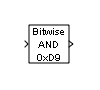# Bitwise Operator

Specified bitwise operation on inputs

• Library:
• Simulink / Logic and Bit Operations

HDL Coder / Logic and Bit Operations

•## Description

The Bitwise Operator block performs the bitwise operation that you specify on one or more operands. Unlike logic operations of the Logical Operator block, bitwise operations treat the operands as a vector of bits rather than a single value.

### Restrictions on Block Operations

The Bitwise Operator block does not support shift operations. For shift operations, use the Shift Arithmetic block.

When configured as a multi-input XOR gate, this block performs modulo-2 addition according to the IEEE® Standard for Logic Elements.

## Ports

### Input

expand all

Input signal, specified as a scalar or vector.

• The NOT operator accepts only one input, which can be a scalar or a vector. If the input is a vector, the output is a vector of the same size containing the bitwise logical complements of the input vector elements.

• For a single vector input, the block applies the operation (except the NOT operator) to all elements of the vector.

• For two or more inputs, the block performs the operation between all of the inputs. If the inputs are vectors, the block performs the operation between corresponding elements of the vectors to produce a vector output.

Data Types: `int8` | `int16` | `int32` | `int64` | `uint8` | `uint16` | `uint32` | `uint64` | `Boolean` | `fixed point`

### Output

expand all

The output signal specified as the output data type, which the block inherits from the driving block, must represent zero exactly. Data types that satisfy this condition include signed and unsigned integer data types.

The size of the block output depends on the number of inputs, the vector size, and the operator you select. If you do not specify a bit mask, the output is a scalar. If you do specify a bit mask, the output is a vector.

Data Types: `int8` | `int16` | `int32` | `int64` | `uint8` | `uint16` | `uint32` | `uint64` | `Boolean` | `fixed point`

## Parameters

expand all

Specify the bitwise logical operator for the block operands.

You can select one of these bitwise operations:

Bitwise OperationDescription

AND

TRUE if the corresponding bits are all TRUE

OR

TRUE if at least one of the corresponding bits is TRUE

NAND

TRUE if at least one of the corresponding bits is FALSE

NOR

TRUE if no corresponding bits are TRUE

XOR

TRUE if an odd number of corresponding bits are TRUE

NOT

TRUE if the input is FALSE (available only for single input)

#### Programmatic Use

 Block Parameter: `logicop` Type: character vector Values: `'AND'`|```'OR' ```|`'NAND'`|```'NOR' ```|`'XOR' ` | `'NOT'` Default: `'AND'`

Select to use the bit mask. Clearing this check box enables Number of input ports and disables Bit Mask and Treat mask as.

#### Programmatic Use

 Block Parameter: `UseBitMask` Type: character vector Values: `'off'`|`'on'` Default: `'on'`

Specify the number of inputs. You can have more than one input ports.

#### Dependency

Clearing the Use bit mask check box enables Number of input ports and disables Bit Mask and Treat mask as.

#### Programmatic Use

 Block Parameter: `NumInputPorts` Type: character vector Values: positive integer Default: `'1'`

Specify the bit mask to associate with a single input. This parameter reads values as hexadecimal values.

You can use the bit mask to set, get, or clear a bit on the input.

To perform a...Set the Operator parameter to...And create a bit mask with...
Bit setORA 1 for each corresponding input bit that you want to set to 1
Bit clearANDA 0 for each corresponding input bit that you want to set to 0
Bit getANDA 1 for each corresponding input bit that you want to get

Suppose you want to set the fourth bit of an 8-bit input vector. The bit mask would be 00010000, which you can specify as `2^4` for the Bit Mask parameter. To clear the bit, the bit mask would be 11101111, which you can specify as `2^7+2^6+2^5+2^3+2^2+2^1+2^0` for the Bit Mask parameter.

Tip

Do not use a mask greater than 53 bits. Otherwise, an error message appears during simulation.

#### Dependency

This parameter is available only when you select Use bit mask.

#### Programmatic Use

 Block Parameter: `BitMask` Type: character vector Values: positive integer Default: `'bin2dec('11011001')'`

Specify whether to treat the mask as a real-world value or a stored integer.

The encoding scheme is `V` = `SQ` + `B`, as described in Scaling (Fixed-Point Designer) in the Fixed-Point Designer™ documentation. `Real World Value` treats the mask as `V`. ```Stored Integer``` treats the mask as `Q`.

#### Dependency

This parameter is available only when you select Use bit mask.

#### Programmatic Use

 Block Parameter: `BitMaskRealWorld` Type: character vector Values: ```'Real World Value'``` | `'Stored Integer'` Default: ```'Stored Integer'```

## Block Characteristics

 Data Types `Booleana` | `fixed point` | `integer` Direct Feedthrough `no` Multidimensional Signals `yes` Variable-Size Signals `no` Zero-Crossing Detection `no` a Bit operations are not recommended for use with Boolean signals.

## Version History

Introduced before R2006a